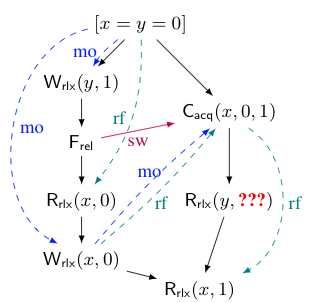Many programmers naively assume that all the behaviours of a concurrent program can be generated by some arbitrary interleaving of its threads. This assumption, however, is incorrect. Due to compiler and/or hardware optimisations, concurrent programs can exhibit a number of behaviours that are not explainable by any interleaving of their threads. The formal study of the exact behaviours concurrent programs can exhibit is a field known as weak memory consistency.

This course will cover the key results in this field, starting with the formal definitions of some important weak memory models, and moving to establishing basic results about them, such as the DRF theorem, and proving the correctness of program transformations within a model or compilation from one model to another. The course will also contain some recent research outcomes, and finish with a set of open research questions. In more detail, the course will cover the following topics:

1. Operational memory models:
• Sequential consistency (SC)
• Total store order (TSO)
• Partial store order (PSO)
• Release-acquire (RA)
2. Axiomatic memory models:
• Basic models: SC, TSO, RA, Coherence
• Comparing axiomatic memory models
• The OOTA problem
3. Fundamental results about memory models:
• The DRF theorem
• Correctness of program transformations
• Relating operational and axiomatic models
4. Resolving the OOTA problem
• Promising semantics
5. Verification of programs under weak memory consistency
• Relaxed separation logic
• Model checking